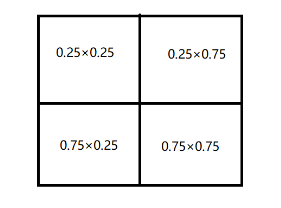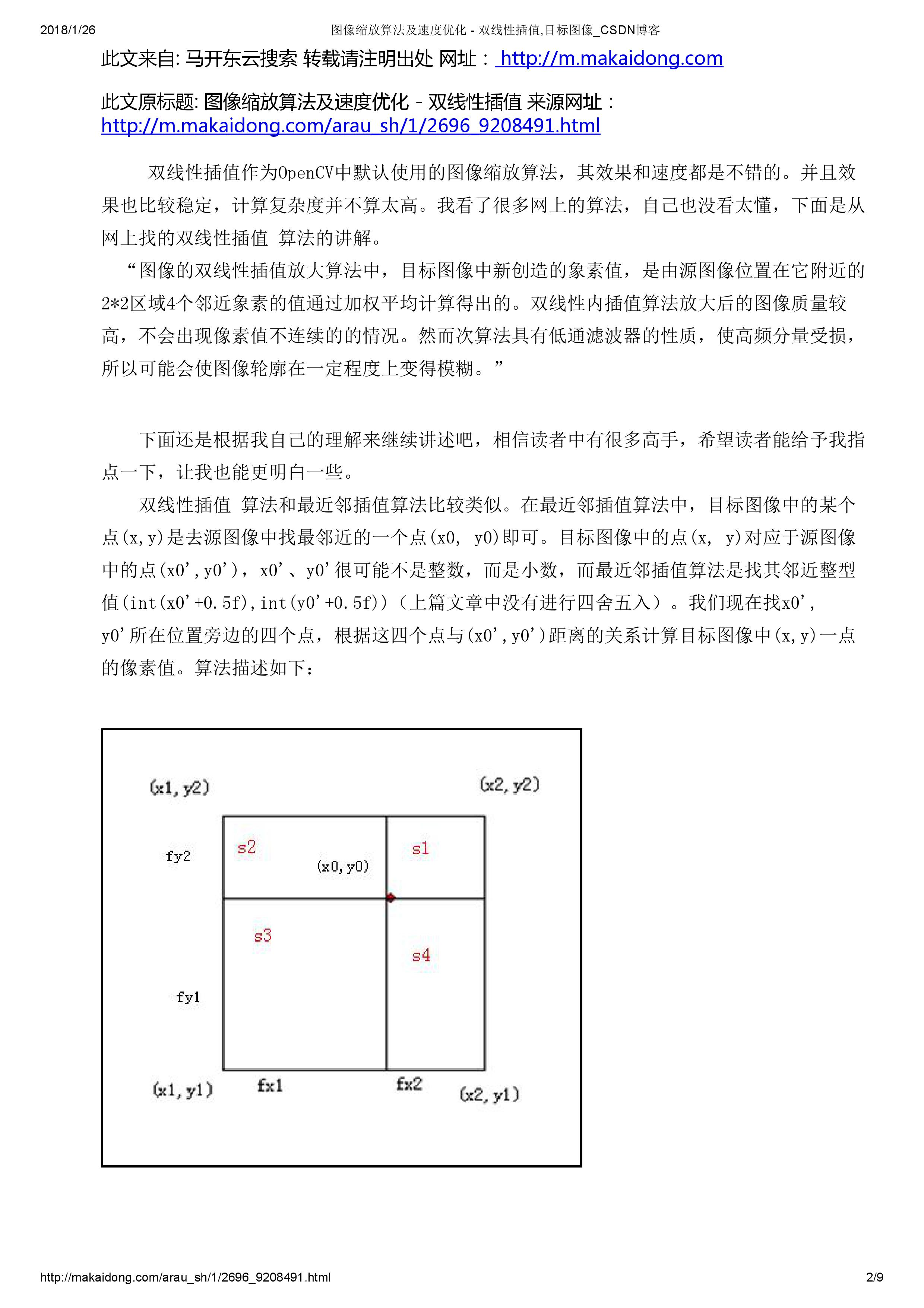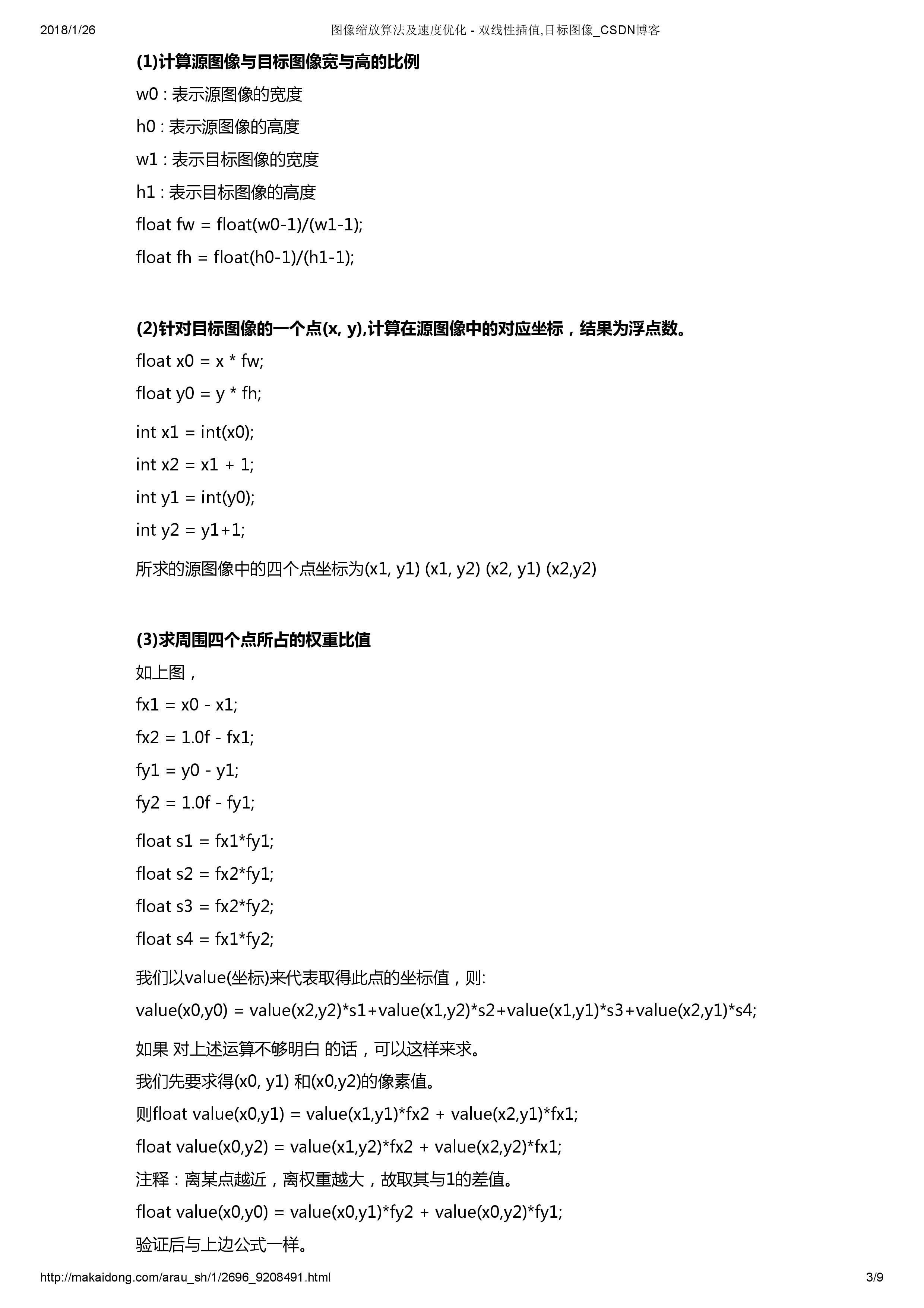• 其中有两种简单又常用的插值算法用来实现图像缩放，分别是最近邻插值算法和双线性插值算法。 最近邻插值算法: 最近邻插值算法的思想十分简单 设原始图像src的高为h，宽为w，图像上像素值为(x,y)。 设目标图像dst...
图像缩放：
resize函数是在OpenCV中经常使用的函数，功能是将一副加载到Mat中的图像调整尺寸或者按照比例进行缩放。
其中有两种简单又常用的插值算法用来实现图像缩放，分别是最近邻插值算法和双线性插值算法。
最近邻插值算法:
最近邻插值算法的思想十分简单
设原始图像src的高为h，宽为w，图像上像素值为(x,y)。
设目标图像dst的高H，宽为W，图像上的像素值为(X,Y)。
那么根据比例缩放的思想有

xX=wW

x

X

=

w

W

$\frac{x}{X}=\frac{w}{W}$  同理，纵向上的像素对应比值为

yY=hH

y

Y

=

h

H

$\frac{y}{Y}=\frac{h}{H}$
那么在考虑目标图像dst上面的像素点(X,Y)对应到原始图像src上面像素点的位置为

(x,y)=(wWX,hHY)

(

x

,

y

)

=

(

w

W

X

,

h

H

Y

)

$(x,y)=(\frac{w}{W}X,\frac{h}{H}Y)$
这里要对

wWX

w

W

X

$\frac{w}{W}X$与

hHY

h

H

Y

$\frac{h}{H}Y$两个数值进行取整，在OpenCV源码当中，是用cvFloor函数取整，即舍去小数部分。
代码：
void InterNearestResize(const Mat &src, Mat &dst, Size &dsize, double fx = 0.0, double fy = 0.0)//原始图形以及缩放比例
{

Size ssize = src.size();//获取矩阵大小
CV_Assert(ssize.area() > 0);//保证矩阵的长宽都大于0

if (!dsize.area())//如果dsize为(0,0)
{
dsize = Size(saturate_cast<int>(src.cols*fx),//satureate_cast防止数据溢出
saturate_cast<int>(src.rows*fy));

CV_Assert(dsize.area());
}
else
{
fx = (double)dsize.width / src.cols;//Size中的宽高和mat中的行列是相反的
fy = (double)dsize.height / src.rows;
}

dst.create(Size(src.cols*fy,src.rows*fx), src.type());//将dst矩阵大小变为想要的尺寸

fx = 1.0 / fx;
fy = 1.0 / fy;
uchar *ps = src.data;
uchar *pd = dst.data;
int channels = src.channels(),x,y,out,in;

for (int row = 0; row < dst.rows; row++)
{
x = cvFloor(row * fx);
for (int col = 0; col < dst.cols; col++)
{
y = cvFloor(col * fy);
for (int c = 0; c < channels; c++)
{
out = (row * dst.cols + col) * channels + c;
in = (x * src.cols + y) * channels + c;
pd[out] = ps[in];
}

//dst.at<Vec3b>(row, col) = src.at<Vec3b>(x, y);
}
}
}
双线性插值算法:
如果原始图像src的大小是3×3，目标图像dst的大小是4×4，考虑dst中(1,1)点像素对应原始图像像素点的位置为(0.75,0.75)，如果使用最近邻算法来计算，原始图像的位置在浮点数取整后为坐标(0,0)。
上面这样粗暴的计算会丢失很多信息，考虑(0.75,0.75)这个信息，它表示在原始图像中的坐标位置，相比较取(0,0)点，(0.75,0.75)貌似更接近(1,1)点，那如果将最近邻算法中的取整方式改为cvRound（四舍五入）的方式取(1,1)点，同样会有丢的信息，即丢失了“0.25”部分的(0,0)点、(1,0)点和(0,1)点。
可以看到，dst图像上(X,Y)对应到src图像上的点，最好是根据计算出的浮点数坐标，按照百分比各取四周的像素点的部分。  如下图：双线性插值的原理相类似，这里不写双线性插值计算点坐标的方法，容易把思路带跑偏，直接就按照比率权重的思想考虑。
将

(wWX,hHY)

(

w

W

X

,

h

H

Y

)

$(\frac{w}{W}X,\frac{h}{H}Y)$写成

(x′+u,y′+v)

(

x

′

+

u

,

y

′

+

v

)

$(x'+u,y'+v)$的形式，表示将

x

x

$x$与y$y$$y$中的整数和小数分开表示

uv

u

v

$uv$分别代表小数部分。
这样，根据权重比率的思想得到计算公式

(X,Y)=(1−u)⋅(1−v)⋅(x,y)+(u−1)⋅v⋅(x,y+1)+u⋅(v−1)⋅(x+1,y)+(u⋅v)⋅(x,y)

(

X

,

Y

)

=

(

1

−

u

)

·

(

1

−

v

)

·

(

x

,

y

)

+

(

u

−

1

)

·

v

·

(

x

,

y

+

1

)

+

u

·

(

v

−

1

)

·

(

x

+

1

,

y

)

+

(

u

·

v

)

·

(

x

,

y

)

$(X,Y)=(1-u)·(1-v)·(x,y)+(u-1)·v·(x,y+1)+u·(v-1)·(x+1,y)+(u·v)·(x,y)$
在实际的代码编写中，会有两个问题，一个是图像会发生偏移，另一个是效率问题。
几何中心对齐：
由于计算的图像是离散坐标系，如果使用

(wWX,hHY)

(

w

W

X

,

h

H

Y

)

$(\frac{w}{W}X,\frac{h}{H}Y)$公式来计算，得到的(X,Y)值是错误的比率计算而来的，即(x+1,y)、(x,y+1)、(x+1,y+1)这三组点中，有可能有几个没有参与到比率运算当中，或者这个插值的比率直接是错误的。  例如，src图像大小是

a×a

a

×

a

$a×a$，dst图像的大小是

0.5a×0.5a

0.5

a

×

0.5

a

$0.5a×0.5a$。
根据原始公式计算

(wW,hH)

(

w

W

,

h

H

)

$(\frac{w}{W},\frac{h}{H})$得到

(2,2)

(

2

,

2

)

$(2,2)$（注意这不是表示点坐标，而是x和y对应的比率）
如果要计算dst点(0,0)对应的插值结果，由于

(2,2)

(

2

,

2

)

$(2,2)$是整数，没有小数，所以最后得到dst点在

(0,0)

(

0

,

0

)

$(0,0)$点的像素值就是src图像上在

(0,0)

(

0

,

0

)

$(0,0)$点的值。然而，我们想要的dst在

(0,0)

(

0

,

0

)

$(0,0)$上的结果是应该是有(0,0)(1,0)(0,1)(1,1)这四个点各自按照0.5×0.5的比率加权的结果。  所以我们要将dst上面的点，按照比率

(wW,hH)

(

w

W

,

h

H

)

$(\frac{w}{W},\frac{h}{H})$向右下方向平移0.5个单位。  公式如下：

(x,y)=(XwW+0.5(wW−1),YhH+0.5(hH−1))

(

x

,

y

)

=

(

X

w

W

+

0.5

(

w

W

−

1

)

,

Y

h

H

+

0.5

(

h

H

−

1

)

)

(x,y)=(X\frac{w}{W}+0.5(\frac{w}{W}-1),Y\frac{h}{H}+0.5(\frac{h}{H}-1))

运算优化：
由计算公式可以得知，在计算每一个dst图像中的像素值时会涉及到大量的浮点数运算，性能不佳。
可以考虑将浮点数变换成一个整数，即扩大一定的倍数，运算得到的结果再除以这个倍数。
举一个简单的例子，计算0.25×0.75，可以将0.25和0.75都乘上8，得到2×6=12，结果再除以

82

8

2

$8^2$，这样运算的结果与直接计算浮点数没有差别。
在程序中，没有办法取得一个标准的整数，使得两个相互运算的浮点数都变成类似“2”和”6“一样的标准整数，只能取一个适当的值来尽量的减少误差，在源码当中取值为

211

2

11

$2^{11}$=2048，即2的固定幂数，最后结果可以通过用位移来表示除以一个2整次幂数，计算速度会有很大的提高。
代码：
void Inter_Linear(const Mat &src, Mat &dst, Size &dsize ,double fx = 0.0, double fy = 0.0)//双线性插值
{
Size ssize = src.size();//获取矩阵大小
CV_Assert(ssize.area() > 0);//保证矩阵的长宽都大于0

if (!dsize.area())//如果dsize为(0,0)
{
dsize = Size(saturate_cast<int>(src.cols*fx),//satureate_cast防止数据溢出
saturate_cast<int>(src.rows*fy));

CV_Assert(dsize.area());
}
else
{
fx = (double)dsize.width / src.cols;//Size中的宽高和mat中的行列是相反的
fy = (double)dsize.height / src.rows;
}

dst.create(dsize, src.type());

double ifx = 1. / fx;
double ify = 1. / fy;

uchar* dp = dst.data;
uchar* sp = src.data;

int iWidthSrc = src.cols;//宽(列数）
int iHiehgtSrc = src.rows;//高(行数)
int channels = src.channels();
short cbufy;
short cbufx;

for (int row = 0; row < dst.rows; row++)
{
float fy = (float)((row + 0.5) * ify - 0.5);
int sy = cvFloor(fy);//整数部分
fy -= sy;//小数部分
sy = std::min(sy, iHiehgtSrc - 2);
sy = std::max(0, sy);

cbufy = cv::saturate_cast<short>((1.f - fy) * 2048);
cbufy = 2048 - cbufy;

for (int col = 0; col < dst.cols; col++)
{
float fx = (float)((col + 0.5) * ifx - 0.5);
int sx = cvFloor(fx);
fx -= sx;

if (sx < 0)
{
fx = 0, sx = 0;
}
if (sx >= iWidthSrc - 1)
{
fx = 0, sx = iWidthSrc - 2;
}

cbufx = cv::saturate_cast<short>((1.f - fx) * 2048);
cbufx = 2048 - cbufx;

for (int k = 0; k < src.channels(); ++k)
{
dp[(row * dst.cols + col) * channels + k] =
(
sp[ ( sy * src.cols + sx ) * channels + k] * cbufx * cbufy +
sp[((sy + 1) * src.cols + sx) * channels + k] * cbufx * cbufy +
sp[(sy * src.cols + (sx + 1)) * channels + k] * cbufx * cbufy +
sp[((sy + 1) * src.cols + (sx + 1)) * channels + k] * cbufx * cbufy
) >> 22;
}
}
}

}
参考博客
对应OpenCV源码部分为  opencv-2.4.9\modules\imgproc\src\imgwarp 部分
展开全文图像缩放
• I am trying to write my own function for scaling up an input image by using the Nearest-neighbor interpolation algorithm. The bad part is I am able to see how it works but cannot find the algorithm it...I am trying to write my own function for scaling up an input image by using the Nearest-neighbor interpolation algorithm. The bad part is I am able to see how it works but cannot find the algorithm itself. I will be grateful for any help.
Here's what I tried for scaling up the input image by a factor of 2:
function output = nearest(input)
[x,y]=size(input);
output = repmat(uint8(0),x*2,y*2);
[newwidth,newheight]=size(output);
for i=1:y
for j=1:x
xloc = round ((j * (newwidth+1)) / (x+1));
yloc = round ((i * (newheight+1)) / (y+1));
output(xloc,yloc) = input(j,i);
end
end
Here is the output after Mark's suggestion解决方案
A while back I went through the code of the imresize function in the MATLAB Image Processing Toolbox to create a simplified version for just nearest neighbor interpolation of images. Here's how it would be applied to your problem:
%# Initializations:
scale = [2 2]; %# The resolution scale factors: [rows columns]
oldSize = size(inputImage); %# Get the size of your image
newSize = max(floor(scale.*oldSize(1:2)),1); %# Compute the new image size
%# Compute an upsampled set of indices:
rowIndex = min(round(((1:newSize(1))-0.5)./scale(1)+0.5),oldSize(1));
colIndex = min(round(((1:newSize(2))-0.5)./scale(2)+0.5),oldSize(2));
%# Index old image to get new image:
outputImage = inputImage(rowIndex,colIndex,:);
Another option would be to use the built-in interp2 function, although you mentioned not wanting to use built-in functions in one of your comments.
EDIT: EXPLANATION
In case anyone is interested, I thought I'd explain how the solution above works...
newSize = max(floor(scale.*oldSize(1:2)),1);
First, to get the new row and column sizes the old row and column sizes are multiplied by the scale factor. This result is rounded down to the nearest integer with floor. If the scale factor is less than 1 you could end up with a weird case of one of the size values being 0, which is why the call to max is there to replace anything less than 1 with 1.
rowIndex = min(round(((1:newSize(1))-0.5)./scale(1)+0.5),oldSize(1));
colIndex = min(round(((1:newSize(2))-0.5)./scale(2)+0.5),oldSize(2));
Next, a new set of indices is computed for both the rows and columns. First, a set of indices for the upsampled image is computed: 1:newSize(...). Each image pixel is considered as having a given width, such that pixel 1 spans from 0 to 1, pixel 2 spans from 1 to 2, etc.. The "coordinate" of the pixel is thus treated as the center, which is why 0.5 is subtracted from the indices. These coordinates are then divided by the scale factor to give a set of pixel-center coordinates for the original image, which then have 0.5 added to them and are rounded off to get a set of integer indices for the original image. The call to min ensures that none of these indices are larger than the original image size oldSize(...).
outputImage = inputImage(rowIndex,colIndex,:);
Finally, the new upsampled image is created by simply indexing into the original image.

展开全文• ## 最近邻插值算法

千次阅读 2018-01-26 16:20:35
转自http://makaidong.com/arau_sh/1/2696_9208491.html转自http://makaidong.com/arau_sh/1/2696_9208491.html
展开全文• 利用最近邻插值法实现图像的缩小与放大.....................................................................................
• 一. 最近邻插值法放大图像： ...最近邻插值算法原理 ↑ 二. 最近邻插值算法流程： 插值法放大图像的第一步都是相同的，计算新图的坐标点像素值对应原图中哪个坐标点的像素值来填充，计算公式为： srcX = ...
一. 最近邻插值法放大图像：
最近邻插值法在放大图像时补充的像素是最近邻的像素的值。由于方法简单，所以处理速度很快，但是放大图像画质劣化明显，常常含有锯齿边缘。最近邻插值法算法原理 ↑

二. 最近邻插值法算法流程：
插值法放大图像的第一步都是相同的，计算新图的坐标点像素值对应原图中哪个坐标点的像素值来填充，计算公式为：
srcX = dstX * (srcWidth/dstWidth)
srcY = dstY * (srcHeight/dstHeight)
其中，src表示旧图，dst表示新图。新图的坐标（dstX,dstY）对应于旧图的坐标（srcX,srcY）。 srcWidth/dstWidth 和 srcHeight/dstHeight 分别表示宽和高的放缩比。
那么问题来了，通过这个公式算出来的 srcX,scrY （旧图坐标）有可能是小数，但是坐标点是不存在小数的，都是整数，得想办法把它转换成整数才行。
不同插值法的区别就体现在 srcX,scrY 是小数时，怎么变成整数去取原图像中的像素值。
最近邻插值法：看名字就很直白，四舍五入选取最接近的整数。这样的做法会导致像素的变化不连续，在新图中会产生锯齿。

三. 实验 python实现最近邻插值算法
from PIL import Image
import matplotlib.pyplot as plt
import numpy as np
import math

# 最近邻插值算法
# dstH为新图的高;dstW为新图的宽
def NN_interpolation(img,dstH,dstW):
scrH,scrW,_=img.shape
retimg=np.zeros((dstH,dstW,3),dtype=np.uint8)
for i in range(dstH-1):
for j in range(dstW-1):
scrx=round(i*(scrH/dstH))
scry=round(j*(scrW/dstW))
retimg[i,j]=img[scrx,scry]
return retimg

im_path='../paojie.jpg'
image=np.array(Image.open(im_path))

image1=NN_interpolation(image,image.shape*2,image.shape*2)
image1=Image.fromarray(image1.astype('uint8')).convert('RGB')
image1.save('out.png')

四. 实验结果：原图 ↑最近邻插值后图像 ↑
可以看到新图中产生了锯齿状的边缘。

五. 参考内容：
https://www.cnblogs.com/wojianxin/p/12515061.html
https://www.jianshu.com/p/4954643f7514
展开全文数字图像处理 图像放大 python实现
• 最近邻插值算法是用图像中已知的像素点填充输出图像，采用像素复制和像素抽样，使原图放大或者缩小若干倍。 假设原图像的宽度和高度分别为 和 ,缩放后的图像的宽度为 和 ,那么宽度和高度的缩放比例分别为： 图片...dst c语言 qt
• 一、算法原理 提出此算法的背景是基于图片的缩放，在图片缩放的过程中，实质上就是将原图像像素矩阵像素值，填到目标图像像素矩阵中，目标图像像素矩阵可能比原图像像素矩阵大（图片放大），也可能小（图片缩小）。...计算机视觉 opencv
• function nearest_neighbor ...%最近邻插值 %输入图像文件及放大倍数 %输出根据放大倍数变化后的新图像 ima=imread(filename); [row,col,color]=size(ima); row_n=round(row*N); col_n=round(col*N); ima_new=zero...
• 这种放大图像的方法叫做最近邻插值算法，这是一种最基本、最简单的图像缩放算法，效果也是最不好的，放大后的图像有很严重的马赛克，缩小后的图像有很严重的失真 。 效果不好的根源就是其简单的最近邻插值方法...
• 插值算法：双三次插值，双线性插值，最近邻插值。 插值方法简述： 假设该图像有n行m列，总像素为nm，x方向和y方向各自扩大kx和ky倍，则： 1.在每行的开头插入u个像素，结尾插入v个像素，在1~(n-1)的每个像素的后面插...计算机视觉
• 最近邻插值 最简单的插值方法，顾名思义，选取距离自己最近的一个点的像素值赋给自己。如图所示： 假设P点为带求像素，其在放大区域的坐标为(X,Y)，其x方向和y方向的放大倍数为kx，ky，通过x/kx...计算机视觉
• 图像常用的插值算法最近邻插值算法双线性插值算法双三次插值(bicubic)算法三种插值算法的优缺点 插值算法是图像缩放中的一项基本且重要的算法；在图像缩放中，输出图像像素点坐标可能对应输入图像上几个像素点之间的...人工智能 深度学习 计算机视觉
• 插值算法最近邻插值、双线性插值 插值算法有很多种，这里列出关联比较密切的三种： 最近邻法(Nearest Interpolation)：计算速度最快，但是效果最差。 双线性插值(Bilinear Interpolation)：双线性插值是用原图像...线性代数 人工智能
• 最近邻插值 与 双线性插值算法 优化迭代 的 0.5 像素之差python opencv 计算机视觉 人工智能 机器学习
• 这就需要插值算法来进行处理，常见的插值算法最近邻插值、双线性插值和三次样条插值。 1.2 最近邻插值 最近邻插值，是指将目标图像中的点，对应到源图像中后，找到最相邻的整数点，作为插值后的输出。 如上图...计算机视觉
• 1.最近邻插值 定义：目标各像素点的灰度值代替源图像中与其最邻近像素的灰度值。 最近临插值算法优点是算法简单，易于实现，但是缺点是由于相邻像素点的像素值相同，容易出现色块现象。 假设源图像（Source）： ...
• 简单来说，用最近邻插值算法实现图像的扩大和缩小任意的尺寸，目标图的任意一点的x,y坐标的像素值取源图最接近x,y坐标的X，Y坐标的像素值，以达到扩大和缩小的目的。 图像也分RGB图和灰度图，下面讨论的是RGB图bmp...
• 这是一种简单的插值算法：不需要计算，在待求象素的四邻象素中，将距离待求象素最近象素灰度赋给待求象素 设i+u, j+v(i, j为正整数， u, v为大于零小于1的小数，下同)为待求象素坐标，则待求象素灰度的值 f(i+u,...
• 图像插值算法包括向上插值和向下插值，向上插值就是对图像进行放大，向下插值就是对图像进行缩小，插值算法在图像预处理过程中经常被使用，通过插值算法，可以将图像尺寸...最近邻插值算法 对于坐标i, 对应于原图...
• 插值主要使用利用到，图像放大、缩小、仿射、透射变换上的。 void cvResize( const CvArr* src, CvArr* dst, int interpolation=CV_INTER_LINEAR );...CV_INTER_NN - 最近邻插值, CV_INTER_LINEAR - 双线性插值 (缺
•图像resize
• 最近邻插值 这是最简单的一种插值算法，当图片放大时，缺少的像素通过直接使用与之最近原有颜色生成，也就是说照搬旁边的像素这样做结果产生了明显可见的锯齿。在待求象素的四邻象素中，将距离待求象素最近的邻灰度...图像处理
• Bayer数据格式转换为RGB数据格式Modelsim仿真，采用最近邻插值算法，转换效果一般，但实现方式最简单。...